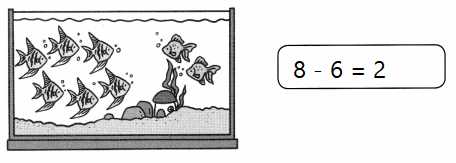# Math in Focus Grade 1 Cumulative Review Chapters 3 and 4 Answer Key

This handy Math in Focus Grade 1 Workbook Answer Key Cumulative Review Chapters 3 and 4 detailed solutions for the textbook questions.

## Math in Focus Grade 1 Cumulative Review Chapters 3 and 4 Answer Key

Concepts and Skills

Look at the pictures. Complete the number sentences.

Question 1.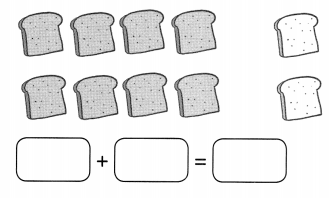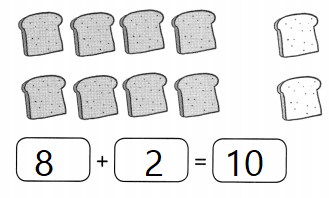Explanation:
8 breads are colored and baked 2 breads are not colored and baked
so, total number of breads baked are = 8 + 2 = 10

Question 2.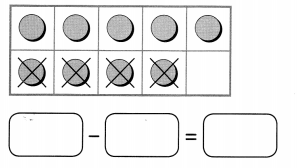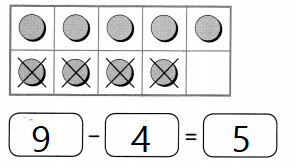Explanation:
In a ten frame there are 9 dots in that 4 are crossed
so, 9 – 4 = 5

Complete the number bonds. Fill in the blanks.

Question 3.
___ + 5 = 10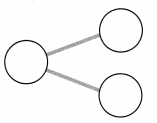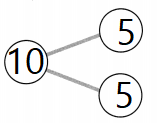Explanation:
The sum of 5 and 5 is 10
that is stated by number bond

Question 4.
8 – 3 = ___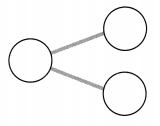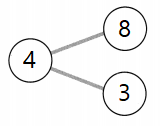Explanation:
The difference of 8 and 3 is 4
that is stated by number bond

Fill in the blanks.

Question 5.
2 more than 8 is ____
10
Explanation:
2 more than 8 is number 10
2 + 8 = 10

Question 6.
3 less than 7 is ___.
4
Explanation:
3 less than 7 is number 4
7 – 3 = 4

Question 7.
_______ is 2 more than 5.
7
Explanation:
2 + 5 = 7
number 7 is 2 more than 5.

Question 8.
________ is 5 less than 10.
5
Explanation:
10 – 5 = 5
number 5 is 5 less than 10.

Find the missing number.

Question 9.
2 + ______ = 8
6
Explanation:
The sum of 2 and 6 is 8
2 + 6 = 8

Question 10.
______ – 6 = 0
6
Explanation:
6 – 6 = 0
The difference of 6 and 6 is 0

Pick three numbers and make a fact family.

Question 11.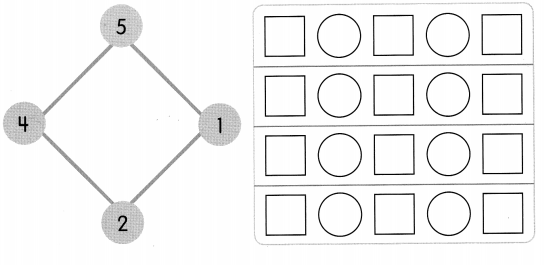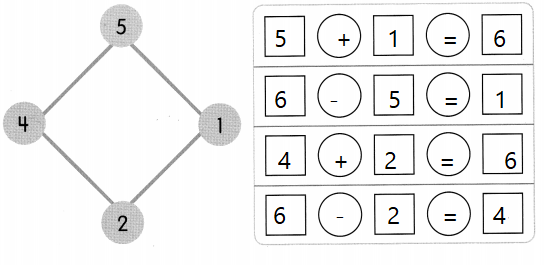Explanation:

An addition fact is defined as the sum of two one-digit addends

Problem Solving
Look at the pictures.
Write an addition or subtraction story.

Question 12.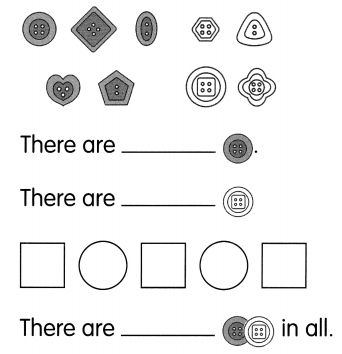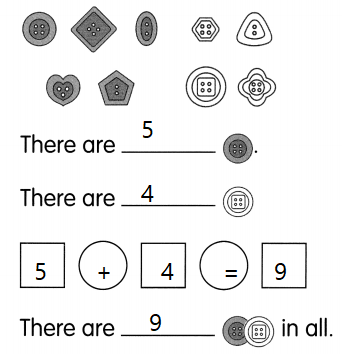Explanation:
There are different shapes of buttons
in them 5 are colored and 4 are non colored
so, total number of buttons are 9
5 + 4 = 9

Question 13.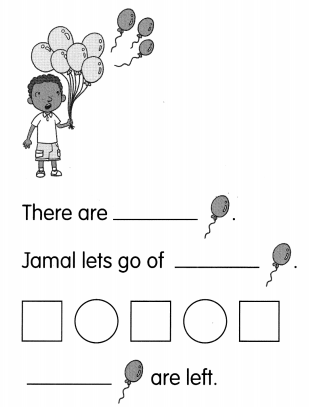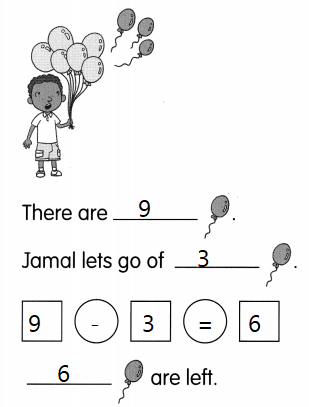Explanation:
Jamel is catching 9 balloons
3 flew away
so, 9 – 3 = 6
6 are left.

Solve.

Question 14.
Ellen has 3 spoons. Her sister gives her 5 spoons. How many spoons does Ellen have now?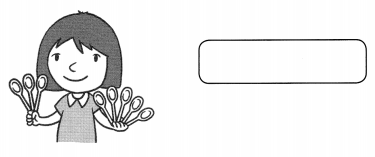Ellen has ___ spoons now.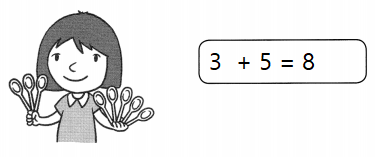Explanation:
Ellen has 3 spoons. Her sister gives her 5 spoons.
Ellen has 8 spoons now.
3 + 5 = 8

Question 15.
There are 8 fish in a fish tank. 6 are angelfish and the rest are goldfish. How many goldfish are there?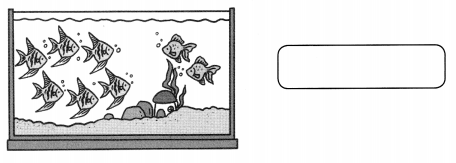There are ___ goldfish.